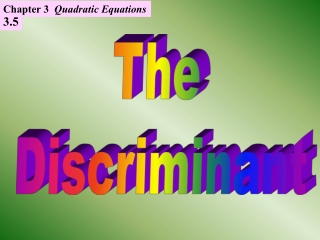DownloadDownload PresentationThe Discriminant

# The Discriminant

Télécharger la présentation## The Discriminant

- - - - - - - - - - - - - - - - - - - - - - - - - - - E N D - - - - - - - - - - - - - - - - - - - - - - - - - - -
##### Presentation Transcript

1. Chapter 3 Quadratic Equations 3.5 The Discriminant

2. TheNature of the Roots The quadratic formula will give the roots of the quadratic equation. From the quadratic formula, the radicand, b2 - 4ac, will determine the Nature of the Roots. • By the nature of the roots, we mean: • whether the equation has real roots or not • if there are real roots, whether they • are different or equal The expression b2 - 4ac is called the discriminant of the equation ax2 + bx + c = 0 because it discriminates among the three cases that can occur.

3. TheNature of the Roots [cont’d] x2 + x - 6 = 0 The value of the discriminant is greater than zero. There are two distinct real roots. x = -3 or 2

4. TheNature of the Roots [cont’d] x2 + 2x + 1 = 0 The value of the discriminantis 0. There are two equalreal roots. x = -1 or - 1

5. TheNature of the Roots [cont’d] 2x2 - 3x + 8 = 0 The value of the discriminant is less than zero. Imaginary roots

6. The Nature of the Roots [cont’d] We can make the following conclusions: If b2 - 4ac > 0, then there are twodifferentreal roots. If b2 - 4ac = 0, then there are twoequalreal roots. If b2 - 4ac < 0, then there are noreal roots. Determine the nature of the roots: x2 - 2x + 3 = 0 4x2 - 12x + 9 = 0 b2 - 4ac = (-2)2 - 4(1)(3) = -8 b2 - 4ac = (-12)2 - 4(4)(9) = 0 The equation has no real roots. There are two equal real roots.

7. Determine the value of k for which the equation x2 + kx + 4 = 0 has a) equal roots b) two distinct real roots c) no real roots a) For equal roots,b2 - 4ac = 0. Therefore, k2 - 4(1)(4) = 0 k2 - 16 = 0 k2 = 16 k = + 4 The equation has equal roots when k = 4 and k = -4. b) For two different real roots,b2 - 4ac > 0. k2 - 16 > 0 k2 > 16 Therefore, k > 4 or k< -4. This may be written as |k| > 4. Which numbers whensquared produce a result that is greater than 16? Which numbers whensquared produce a result that is less than 16? c) For no real roots, b2 - 4ac < 0. k2 < 16 Therefore, -4 < k < 4. This may be written as |k| < 4.